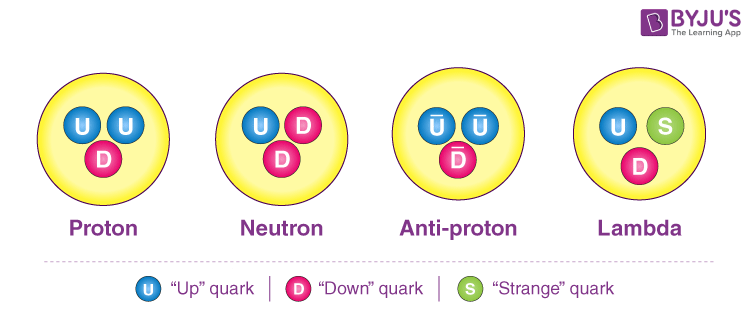# Quark

When you deal with nuclear physics, we usually come across matter that is made up of particles such as protons, neutrons, and electrons. These particles are made up of subatomic particles called Quarks.

## What is Quark?

Quark is a fundamental constituent of matter and is defined as an elementary particle. These quarks combine to produce composite particles called hadrons, the most stable of which are neutrons and protons which are the components of atomic nuclei. We can define quark as:

A quark is a type of elementary particle and a fundamental constituent of matter.

 Theorized: George Zweig (1964); Murray Gell-Mann (1964) Types: 6 (up, down, strange, charm, bottom, and top) Spin: 1⁄2 Baryon number: 1⁄3 Symbol: q

### Examples of Quarks

Protons consist of two up quarks and one down quark, whereas a neutron is made up of two down quarks and one up quark.

Quarks cannot exist independently but as a constituent part of the matter. Its Standard Theoretical Model is based on the conceptual framework. It describes all the known elementary particles. Also, it describes the unobserved particles.

The three primary quark types are mentioned below.

1. Up
2. Down
3. Strange

The antiparticles that correspond to every flavour of quarks are known as Antiquarks. Antiquarks have the same mass, same mean lifetime, and same spin corresponding to quarks, but other properties like electric charge and other charges have opposite signs.

Twelve fundamental particles, i.e. six quarks and six leptons (the other type) are the basic building blocks of everything in the universe. Quark and leptons are distinguished based on flavours. The six types are namely: up, down, top, bottom, strange, and charm.## Types of Quarks

The six types are namely:

 Up Bottom Down Strange Top Charm

### Up Quark

Up quarks are the lightest among all the quarks. They have maximum stability due to the lowest mass.

• The symbol used is u, and its antiparticle is denoted by u.
• The mass of the Up quark ranges from 1.7 – 3.1 MeV / c2.
• Its electronic charge is +2/3 e.

### Down Quark

The down quark comes next to up quarks regarding its light mass. Therefore, it also has high stability.

• Down quark is denoted by d, and its antiparticle is denoted by d.
• The mass of the down quark ranges from 4.1 – 5.7 MeV / c2.
• Its electric charge is -1/3 e.

### The Strange Quark

The strange quark comes under the third lightest among all.

• Strange quark is denoted by s, and its antiparticle is denoted by S.
• Its electric charge is -1/3 e.

### The Charm Quark

The meson which is called a J/Psi particle is an example of the charm quark.

• Charm Quark is denoted by C, and its antiparticle is denoted by C.
• The electric charge is a quark of +2/3 e.

### The Top Quark

The Top quark is denoted by t and its antiparticle is denoted by t.

• The mass of the top quark is 172.9 – 1.5 GeV/c2.
• Its electric charge is +2/3.

### The Bottom Quark

The bottom quark is symbolized by b and its antiparticle is denoted by b.

• The mass of the bottom quark is approximately 4.1 GeV/c2.
• Its electric charge is -1/3 e.

## Watch the video and understand matter and its characteristics.## Frequently Asked Questions – FAQs

Q1

### What is a quark?

A quark is a type of elementary particle and a fundamental constituent of matter.
Q2

### What is the electronic charge of the up quark?

The electronic charge of the up quark is 2/3 e.
Q3

### What are the types of quarks?

Types of quarks are:
• Up
• Down
• Top
• Bottom
• Strange
• Charm
• Q4

### How is a down quark and its antiparticle denoted?

Down quark is denoted as d, and its antiparticle is denoted by d.
Q5